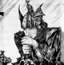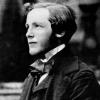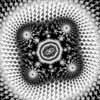1.## boo

Senior Members

8

• ### Content Count

62

2.## studiot

Senior Members

2

• ### Content Count

12668

3.## Endy0816

Senior Members

1

• ### Content Count

3108

4.## Mordred

Resident Experts

1

8048

## Popular Content

Showing content with the highest reputation on 08/25/19 in all areas

1. 2 points## hijack from Pascal's wager and atheism (split from What made you stop believing in God?)

and this is coming from the person defending religion? dear god! im through with this. its going nowhere
2. 2 points## hijack from Pascal's wager and atheism (split from What made you stop believing in God?)

wow, you really cant bring yourself to just give a straight answer......not even a one word answer. its your argument, make it. there is plenty of bad with the little good, that is the point. and who decides that the lie is a good thing? the liar or the person being lied to?
3. 1 point## hijack from Pascal's wager and atheism (split from What made you stop believing in God?)

Now that's me trying to be clever, because... "No-one expects the Spanish inquisition"... I may have failed, but it made me giggle.. Have the magic man, don't have the magic man... I don't give a shit, why do you? That reminds me... You do know that an extraordinary claim requires extraordinary evidence, don't you? what is up with you? you are acting very immature now I'm entitled to my view, and you to yours. we can rationalize it maturely if you want. I don't object to other people being religious. you have either misinterpreted me, or you are taking offence to my views and are overreacting.
4. 1 point
Perhaps a little more detail is needed here ? What is the fluid represented by the blue lines? I am guessing the following: Your text is about the rate of flow or speed of flow of something like water out of a hole in the bottom of the tank (called orifice discharge), using Bernoulli's theorem and the continuity equation. I expect that somewhere your text indicates that atmospheric pressure is sensibly the same at the top and bottom of the tank, because 'h' will be very small compared to the height of the air column causing p0 , the pressure at A1. So the discharge will depend only on the head (h) and density of the fluid in the tank.
5. 1 point
THANK You for your comment !!!... I am only asking if a "Fullerite C24" can be done by Natur (or by Humans) having in Mind that the "Cubic Fullerite C24" is made by scientists !!!... ( Is there some reason that prevent the construction of "Fullerite C24" ???... ) https://link.springer.com/article/10.1134/1.1649442
6. 1 point
By atmospheric pressure they mean the pressure of the Earth's atmosphere at the lowest point. I like to imagine a column of air above me weighing me down. Weight(force) / Area = Pressure
7. 1 point
Ok, http://www.einsteins-theory-of-relativity-4engineers.com/LightCone7/LightCone.html the equality with all $\Omega$ allow to show it. ( and $\Omega_0=1$ is in input parameters ) we gave both a part of the solution : and so, for $\Lambda$, it's $\Omega_{\Lambda,H}$ in relation to $\Omega_{\Lambda,H0}$ which reflects the evolution of $H$, the latter also affecting the critical density, both by $H^2$ ... … certainly, with the exception ( very probably) of the "young" universe. Did I succeed to answer the first question of last quote in this message ( I had miss something ?) ? For 2*, in the near past period and for the whole future, this affects $H^2/H0^2$ by about 0.1%. It is reasonable to say that, in these cases, the impact is negligible, but it cannot be denied.
8. 1 point## Pascal's wager and atheism (split from What made you stop believing in God?)

Might want to increase your fibre intake.
9. 1 point## Pascal's wager and atheism (split from What made you stop believing in God?)

another deflection. fair enough.
10. 1 point## Pascal's wager and atheism (split from What made you stop believing in God?)

the only one who can spell out your views is you. if you want to engage in a conversation as an equal, then you would afford me that much. Fry is funny i like him, he has said some things that would put him very much in the "bad atheist" category acccording to some people.Things I would not even say. In fact, I think it was partly thanks to him that the anti blasphemy law was finally gotten rid of in Ireland. vHe was criticizing God on a television Interview and someone reported his anti God comments to the Irish Police. The police never followed it up, presumably because the will wasn't there to enforce such a draconian law. shortly afterwards we had a referrendum to get rid of the law, just a few months ago actually. Yep. 2019, not the 1600's. 2019
11. 1 point## Pascal's wager and atheism (split from What made you stop believing in God?)

oh, so you are still talking to me? whatever for, im not sure. I cant see the second video, it is blocked in my country. the first one is funny, so im a "bad" atheist am I? LOL I just gave my honest account. go ahead and explain why its bad, tell me the alternative (without lying about what i said or being unnecessarily patronizing) but i doubt you will at this point, that doesn't seem to be your goal.
12. 1 point## FLUIDS (Pressure in a fluid of uniform density

Timo's posh explanation is just fine but try this simplified one. Looking at the diagram I see that upwards is defined positive. Looking at your equation and the diagram pA - (p+dp) A - ρgAdy So I see that the arrow on the underside is pointing up so pA is positive. But on the top, p and dp point downwards so are negative so (p+dp) is negative on the top dy is positive and g is negative so the third term is also negative. does this help?
13. 1 point
OK so you want particles and and antiparticles popping in and put. So let's use the field treatment of QFT. Now the positive frequency modes form the annihilation operators for particles with the negative frequency modes for the creation operators of anti particles. $\hat{a}^\dagger (\vec{k}) \hat{a}(\vec{k})$ the former is creation the latter annihilation operators for particles For antiparticles $\hat{b}^\dagger (\vec{k}) \hat{b}(\vec{k})$ Hence $\hat{a}^\dagger (\vec{k})$ creates a particle of energy $\hbar\omega$ and momentum $\hbar k$ same applies to the antiparticles Now with the above you sum up the positive frequency parts with the negative frequency parts. $\hat{\psi}(x)=\int\frac {d^3k}{(2\pi)^{\frac {3}{2}}\sqrt{2\omega_k}}$$\hat{a}(\vec{k})e^{-i(\omega_kx^0-\vec{k}\cdot\vec{x})}$$+\vec {b}^\dagger(\vec{k})e^{-i(\omega_kx^0-\vec{k}\cdot\vec{x})}$ How's that for a start to your model you now have the a scalar spacetime complex field of particles and antiparticles. Now accepting that baryogenesis leaves a slight higher density of positive frequency parts (cause unknown) I will let you think about this in terms of the energy density values for different observers for the Unruh effect in your link above. Naturally the mass density valued will vary accordingly to field potential which will affect the path integrals described by the Feynman lines you referred to. (I am going to up vote you +1 for coming up with a viable speculation model though we can improve your descriptive as we go ) Now continuing from above a complex field has an adjoint. $\hat{\psi}^\dagger(x)=\int\frac {d^3k}{(2\pi)^{\frac {3}{2}}\sqrt{2\omega_k}}$$\hat{a}(\vec{k})e^{-i(\omega_kx^0-\vec{k}\cdot\vec{x})}$$+\vec {b}^\dagger(\vec{k})e^{-i(\omega_kx^0-\vec{k}\cdot\vec{x})}$ Now assuming you want bosons for an uncharged field. We can incorporate the Pauli exclusion symmetry to the Bose Einstein statistics So first we have normalize the vacuum to unity. In Dirac notation $\langle 0|0\rangle=1$. The ket $|\rangle$ is the initial state the bra $\langle |$ is the final state. So we need to compute the normalization to an arbitrary state. $|\vec{k}\rangle$ for that we need the inner product $\langle\vec{k}|\acute{\vec{k}}\rangle$ So $\hat{a}^\dagger (\vec{k})|0\rangle=|\vec{k}\rangle$ with adjoint $\langle 0|=\langle\vec{k}|\hat{a}(\vec{k})$ Without going through all the steps $\langle\vec{k}|\acute{\vec{k}}\rangle=\delta(\vec{k}-\acute{\vec{k}})$ Now each k state represents the momentum of a single particle. If they are bosons then the following relation holds $|\vec{k_1},\vec{k_2}\rangle=|\vec{k_2},\vec{k_1}\rangle$ Now you have a complex spin zero boson field in momentum space. Next lesson we apply thus to the four momentum of GR. As we're dealing with a complex scalar boson field of particles/antiparticles let's start with the Minkowskii metric $ds^2=-c^2dt^2+dx^2+dy^2+dz^2=\eta_{\mu\nu}dx^{\mu}dx^{\nu}$ Apply coordinate notation $(x^0,x^1,x^2,x^3x^4)=(ct,x,y,z)=x^\mu$ Where the indice range is 0 to 3. Four momentum is given here https://en.m.wikipedia.org/wiki/Four-momentum In the format I have provided using a particle number operator I will just latex the positive frequency modes as the negative frequency modes simply switch a to b. $\hat{N}=\hat{a}^\dagger (\vec{k})\hat{a}(\vec{k})$ The Hamilton is $\hat{H}=\int d^3k\omega_k [\hat {N}(\vec{k})+\frac{1}{2}]$ includes the harmonic oscillator. The field momentum $\hat{P}=\int k\vec{k}[\hat {N}(\vec{k})+\frac{1}{2}]$ Now there is an interesting consequence of this when you compute the energy of the field...it is related to the energy of the harmonic oscillator. Can you guess what it is ? I will show the answer tomorrow. PS to OP I hope your descriptive above needs considerable work however you have several details with accuracy that although poorly described are applicable. So I hope you don't mind if I run the modelling gauntlet with the basis of particle/antiparticle creation and annihilation and it's effects. I will get to your network analogy later on as I can employ that analogy
14. 1 point## Hey Science people. Caught myself lurking here more and more and I realized how much

Hey Science people. Caught myself lurking here more and more and I realized how much I enjoy rational people with critical thinking skills, so i decided to log in to say thanks. Shout-out to any of the old regular posters lurking out there.
15. -1 points## Pascal's wager and atheism (split from What made you stop believing in God?)

I am a monkey, I'm programmed too toss...
16. -1 points## Pascal's wager and atheism (split from What made you stop believing in God?)

17. -1 points## hijack from Pascal's wager and atheism (split from What made you stop believing in God?)

Because, sometimes your Spanish saying is true. Because you don't seem to get it, and on my map that leads to 'frustration' (an abandoned town in the middle of the bad lands)...
×